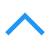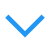# 卷积感受野计算

## 感受野（receptive field）

CNN中，某一层输出结果中一个元素所对应的输入层的区域大小.

## 感受野计算

### 从后往前

output field size = ( input field size - kernel size + 2 × padding ) / stride + 1,变形之后得到input field size = （output field size - 1）× stride - 2 × padding + kernel size。因此可以通过后式从最后一层到第一次级联后计算在原图上的感受野。计算感受野时不需要考虑padding(因为我们不是在计算具体有边界的映射区域,而是计算抽象的大小):

RF = 1 #待计算的feature map上的感受野大小
for layer in （top layer To down layer）:
RF = ((RF -1)* stride) + kernel size


### 从前往后

$l_k = l_{k-1} + ((f_k - 1) * \prod_{i=1}^{k-1}s_i)$

$l_k$ 是第k层的每个点的感受野, $f_k$是第k层卷积核的大小(宽或高, 并假设宽高相等), $s_i$是第i层卷积的stride, 在第k层的感受野比第k-1层的感受野大 $(f_k−1)\times S_{k−1}=(f_k - 1) \times \prod_{i=1}^{k-1}s_i$.

### 举例

posted @ 2018-07-16 17:31  康行天下  阅读(3851)  评论(0编辑  收藏  举报